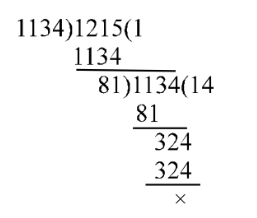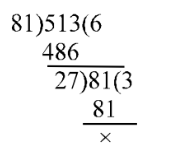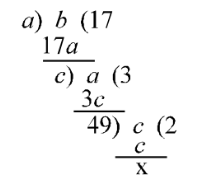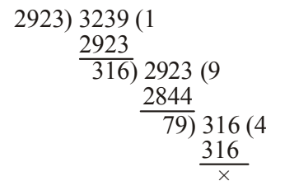## LCM and HCF

#### LCM and HCF

1. Find the HCF of 513, 1134 and 1215.

1. At first, we find out the HCF of 1134 and 1215∴ HCF of 1134 and 1215 is 81.
∴ Required HCF = HCF of 513 and 81.

##### Correct Option: A

At first, we find out the HCF of 1134 and 1215∴ HCF of 1134 and 1215 is 81.
∴ Required HCF = HCF of 513 and 81.∴ HCF of given numbers = 27

1. In finding the HCF of two numbers by division method, the last divisor is 18 and quotients are 2, 7 and 3. Find the numbers.

1. Here , Last divisor = 18 and quotient = 3
∴ Dividend = 18 × 3 = 54
Now, divisor = 54, quotient = 7 and remainder = 18
∴ Dividend = 7 × 54 + 18 = 378 + 18 = 396
Now, divisor = 396, quotient = 2 and remainder = 54
∴ Dividend = 2 × 396 + 54 = 792 + 54 = 846

##### Correct Option: C

Here , Last divisor = 18 and quotient = 3
∴ Dividend = 18 × 3 = 54
Now, divisor = 54, quotient = 7 and remainder = 18
∴ Dividend = 7 × 54 + 18 = 378 + 18 = 396
Now, divisor = 396, quotient = 2 and remainder = 54
∴ Dividend = 2 × 396 + 54 = 792 + 54 = 846
Hence, the required numbers are 396 and 846.

1. In finding the HCF of two numbers by division method, the last divisor is 49 and the quotients are 17, 3, 2. Find the two numbers.

1. Here, the last divisor = 49 and quotient = 2
or, Symbolically the process of finding the HCF by division method can be shown in this way.the dividend (c) = 49 × 2 = 98
Now, divisor = 98,
quotient = 3 and remainder = 49
∴ Dividend (a) = 98 × 3 + 49 = 294 + 49 = 343
Again, divisor = 343, quotient = 17 and remainder = 98
∴ Dividend (b) = 343 × 17 + 98 = 5831+ 98 = 5929
Thus, two numbers are 343 and 5929.
Second method to solve this question :

 a b c 49 Remainders → 17 3 2

##### Correct Option: B

Here, the last divisor = 49 and quotient = 2
or, Symbolically the process of finding the HCF by division method can be shown in this way.the dividend (c) = 49 × 2 = 98
Now, divisor = 98,
quotient = 3 and remainder = 49
∴ Dividend (a) = 98 × 3 + 49 = 294 + 49 = 343
Again, divisor = 343, quotient = 17 and remainder = 98
∴ Dividend (b) = 343 × 17 + 98 = 5831+ 98 = 5929
Thus, two numbers are 343 and 5929.
Second method to solve this question :

 a b c 49 Remainders → 17 3 2

Let two numbers are a and b.
c = 2 × 49 = 98
b = 3c + 49 = 3 × 98 + 49 = 343
a = 17b + c = 17 × 343 + 98 = 5929

1. What is the greatest number that will divide 2930 and 3246 that will leave 7 as remainder in each case.

1. Obviously, the greatest number will divide completely the numbers (2930 – 7) and (3246 – 7),
i.e., 2923 and 3239.
Hence, the greatest number will be the HCF of 2923 and 3239.##### Correct Option: A

Obviously, the greatest number will divide completely the numbers (2930 – 7) and (3246 – 7),
i.e., 2923 and 3239.
Hence, the greatest number will be the HCF of 2923 and 3239.∴ HCF = 79
Hence, the required number = 79

1. The numbers 11284 and 7655 when divided by a number of three digit, leave the same remainder. Find the number of three digits.

1. Let the remainder in each case be t.
Then, (11284 – t) and (7655 – t) are exactly divisible by that three digit number.
Hence, their difference is [(11284 – t ) – (7655 – t ] = 3629 will also be exactly divisible by that three digit number. In other words that divisor will be a factor of 3629.
Now, 3629 = 19 ×191

##### Correct Option: D

Let the remainder in each case be t.
Then, (11284 – t) and (7655 – t) are exactly divisible by that three digit number.
Hence, their difference is [(11284 – t ) – (7655 – t ] = 3629 will also be exactly divisible by that three digit number. In other words that divisor will be a factor of 3629.
Now, 3629 = 19 ×191
Since both 19 and 191 are prime numbers, the three digit number is 191.
Hence, the required number = 191